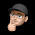## Friday, November 30, 2012

### Converting a Hex String to a Byte Array in Scala

Here's a Scala method that converts a hex string to an array of bytes. This is useful when needing to encrypt a hex string, because most of the crypto libraries operate on byte arrays.

```def hexStringToByteArray(s : String) = {
val len = s.length();
var data = new Array[Byte](len / 2)
var i = 0

while (i < len) {
val b = (Character.digit(s.charAt(i), 16) << 4) +
(Character.digit(s.charAt(i+1), 16))

data(i / 2) = b.asInstanceOf[Byte]

i+=2
}

data
}
```

1.Asserted hex strings always have an even length, the conversion could be written without mutable data:

def hexStringToByteArray(s: String) = s.grouped(2).map(cc => (Character.digit(cc(0),16) << 4 | Character.digit(cc(1),16)).toByte).toArray

I assume, there are many things which can be improved. It depends on the context and the restrictions where the method is to be used.
Kind regards JP

2.